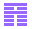# The CLP(OIH) Language

Ole Ildsgaard Hougaard

February 1998

### Abstract:

Many type inference problems are different instances of the same constraint satisfaction problem. That is, there is a class of constraints so that these type inference problems can be reduced to the problem of finding a solution to a set of constraints. Furthermore, there is an efficient constraint solving algorithm which can find this solution in polynomial time.

We have shown this by defining the appropriate constraint domain, devising an efficient constraint satisfaction algorithm, and designing a constraint logic programming language over the constraint domain. We have implemented an interpreter for the language and have thus produced a tool which is well-suited for type inference problems of different kinds.

Among the problems that can be reduced to our constraint domain are the following:

• The simply typed-calculus.
• The-calculus with subtyping.
• Arbadi and Cardelli's Object calculus.
• Effect systems for control flow analysis.
• Turbo Pascal.
With the added power of the constraint logic programming language, certain type systems with no known efficient algorithm can also be implemented -- e.g. the object calculus with selftype.

The programming language thus provides a very easy way of implementing a vast array of different type inference problems

Available as PostScript, PDF.BRICS WWW home page